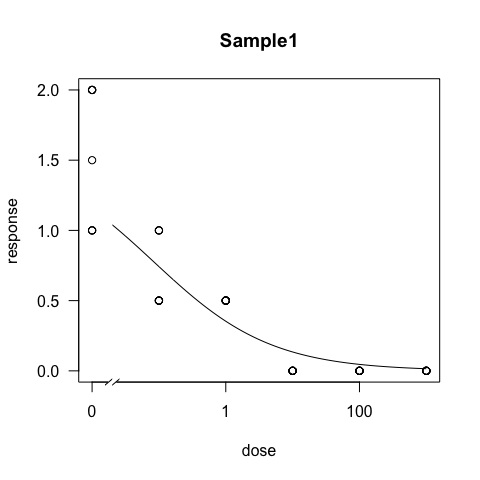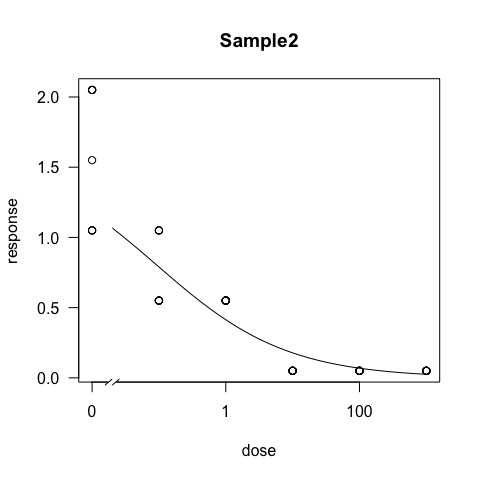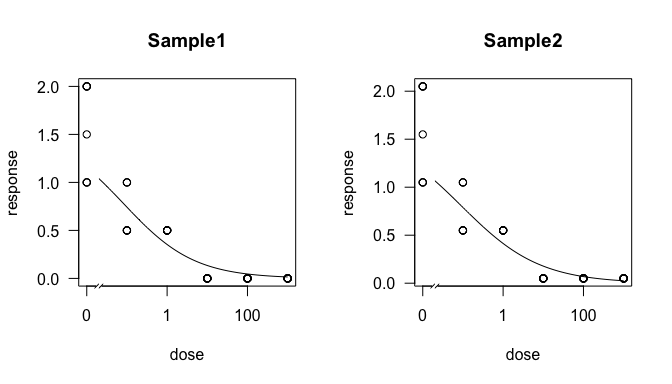# Welcome

## Introduction

The package EZEC stands for Easy Effective Concentration. This package was designed to be a simple wrapper for the functions drm() and ED() in the drc package. It provides the function EC_table() that will first fit a model to your data and then calculate effective concentrations from that model over several samples arranged in a data frame. In this tutorial, I will give a couple of short examples of how you can analyze your data. To get help with any functions in the package, simply type help("function.name") and replace function.name with the name of the function you want help for.

## Credit

As this package is simply a wrapper to two functions from the drc package, please cite the drc package and indicate the version number you used:

packageVersion("drc")
##  '2.5.12'
citation("drc")
##
## To cite the package drc in publications use:
##
##   Ritz, C. & Streibig, J. C. (2005) Bioassay Analysis using R. J.
##   Statist. Software, Vol 12, Issue 5.
##
## A BibTeX entry for LaTeX users is
##
##   @Article{,
##     title = {Bioassay Analysis using R},
##     author = {C. Ritz and J. C. Streibig},
##     journal = {Journal of Statistical Software},
##     volume = {12},
##     issue = {5},
##     year = {2005},
##     url = {http://www.bioassay.dk},
##   }

# Data Format

Your data must be in a format that encodes one observation per row. You can see an example of this in the dummydata data set:

library("ezec")
data("dummydata", package = "ezec")
head(dummydata) # the function head means "look at the top of the object"
##        ID strain      fung dose rep response
## 1 Sample1      a Metalaxyl  0.0   1      1.5
## 2 Sample1      a Metalaxyl  0.0   2      1.0
## 3 Sample1      a Metalaxyl  0.1   1      0.5
## 4 Sample1      a Metalaxyl  0.1   2      1.0
## 5 Sample1      a Metalaxyl  1.0   1      0.5
## 6 Sample1      a Metalaxyl  1.0   2      0.5

You can see that this contains the columns ID, strain, fung, dose, rep, response. The only important columns here are the ID, dose, and response columns.

To import your own data from csv text file, you should use the function read.table() to import it into R. If you want to import data from an xlsx file, you can use the function read_excel() from the readxl package.

# Data Analysis

You can analyze your data simply by running EC_table() with your data and supplying a formula to describe what you want to analyze in the form of response_variable ~ explanitory_variable. In our example of “dummydata”, our response variable is “response” and our explanitory variable is “dose”.

library("ezec")
data("dummydata", package = "ezec")
res <- EC_table(dummydata, form = response ~ dose)print(res)
## # A tibble: 2 × 7
##    sample  Estimate.10        SE.10 Estimate.50      SE.50 Estimate.90
##     <chr>        <dbl>        <dbl>       <dbl>      <dbl>       <dbl>
## 1 Sample1 0.0009539812 0.0011786368  0.08287674 0.04098527    7.199884
## 2 Sample2 0.0006601386 0.0008750109  0.09240879 0.04713886   12.935744
## # ... with 1 more variables: SE.90 <dbl>

If you want to have all the plots plotted in one window, you can use the par() function:

par(mfrow = c(1, 2)) # set window to have 1 row and two columns
EC_table(dummydata, form = response ~ dose)## # A tibble: 2 × 7
##    sample  Estimate.10        SE.10 Estimate.50      SE.50 Estimate.90
##     <chr>        <dbl>        <dbl>       <dbl>      <dbl>       <dbl>
## 1 Sample1 0.0009539812 0.0011786368  0.08287674 0.04098527    7.199884
## 2 Sample2 0.0006601386 0.0008750109  0.09240879 0.04713886   12.935744
## # ... with 1 more variables: SE.90 <dbl>
par(mfrow = c(1, 1)) # reset the window

## Saving your results to a file

If you want to save your results to a file that you can format for a manuscript, you can use the write.table() functon:

write.table(res, file = "dummy_results.csv", row.names = FALSE, sep = ",")

## Output: model summary

You can also have the output be a summary of the model for each sample as a list

EC_table(dummydata, form = response ~ dose, plot = FALSE, result = "summary")
## $Sample1 ## ## Model fitted: Log-logistic (ED50 as parameter) with lower limit at 0 (3 parms) ## ## Parameter estimates: ## ## Estimate Std. Error t-value p-value ## Slope:(Intercept) 0.492158 0.096463 5.102022 0.0000 ## Upper Limit:(Intercept) 1.556544 0.084577 18.403838 0.0000 ## ED50:(Intercept) 0.082877 0.040985 2.022111 0.0491 ## ## Residual standard error: ## ## 0.2379276 (45 degrees of freedom) ## ##$Sample2
##
## Model fitted: Log-logistic (ED50 as parameter) with lower limit at 0 (3 parms)
##
## Parameter estimates:
##
##                          Estimate Std. Error   t-value p-value
## Slope:(Intercept)        0.444645   0.085522  5.199200  0.0000
## Upper Limit:(Intercept)  1.607358   0.083651 19.215124  0.0000
## ED50:(Intercept)         0.092409   0.047139  1.960353  0.0562
##
## Residual standard error:
##
##  0.2355344 (45 degrees of freedom)

## Output: model

You can also choose to have the output be the model itself

EC_table(dummydata, form = response ~ dose, plot = FALSE, result = "model")
## $Sample1 ## ## A 'drc' model. ## ## Call: ## drc::drm(formula = form, data = x, fct = MODEL(names = mod.names), na.action = na.omit) ## ## Coefficients: ## Slope:(Intercept) Upper Limit:(Intercept) ED50:(Intercept) ## 0.49216 1.55654 0.08288 ## ## ##$Sample2
##
## A 'drc' model.
##
## Call:
## drc::drm(formula = form, data = x, fct = MODEL(names = mod.names),     na.action = na.omit)
##
## Coefficients:
##       Slope:(Intercept)  Upper Limit:(Intercept)         ED50:(Intercept)
##                 0.44464                  1.60736                  0.09241